• 1KB weixin_42685438 2021-10-02 12:09:57
•4星
22KB weixin_42696333 2021-09-11 01:23:26
• 希尔伯特黄变换代码matlab 希尔伯特黄变换

2KB weixin_43967360 2021-06-17 21:29:00
• 希尔伯特黄变换在含噪声语音信号处理中的应用 希尔伯特黄

4.69MB qq_34254316 2018-03-10 09:04:47
•3星
3KB zjq1347911 2017-12-12 19:09:22
• 688KB weixin_38666300 2020-07-02 17:34:19
• 10.76MB weixin_38604330 2021-02-06 06:22:07
• 47KB ximinc 2018-03-05 00:24:19
• 767KB sophyabuaa 2018-12-26 11:02:58
• HHT希尔伯特黄变换 MATLAB工具箱 HHT MATLAB

3.82MB mlxx125 2018-11-17 12:53:01
• 168KB q191201771 2021-03-18 22:30:16
• 语音信号处理 | 傅里叶变换、短时傅里叶变换、小波变换、希尔伯特变换、希尔伯特黄变换 信号处理 数字信号处理 matlab 小波分析 希尔伯特变换

在信号处理领域，存在诸多变换，比如标题中的五个变换。本文将对这五个变换进行介绍和比较。在开始之前，我们需要先理清什么是平稳信号，什么是非平稳信号。 我们知道，自然界中几乎所有信号都是非平稳信号，比如...

在信号处理领域，存在诸多变换，比如标题中的五个变换。本文将对这五个变换进行介绍和比较。在开始之前，我们需要先理清什么是平稳信号，什么是非平稳信号。

我们知道，自然界中几乎所有信号都是非平稳信号，比如我们的语音信号就是典型的非平稳信号。那么何谓平稳信号和非平稳信号呢？一个通俗的理解即，平稳信号在不同时间得到的采样值的统计特性(比如期望、方差等)是相同的，非平稳信号则与之相反，其特性会随时间变化。在信号处理中，这个特性通常指频率。

通常傅里叶变换只适合处理平稳信号，对于非平稳信号，由于频率特性会随时间变化，为了捕获这一时变特性，我们需要对信号进行时频分析，就包括短时傅里叶变换小波变换希尔伯特变换希尔伯特黄变换这几种变换。以下逐一进行分析介绍。

傅里叶变换(Fourier Transform, FT)

首先考虑一个连续信号 f ( t ) f(t) 的傅里叶变换和它的反变换，如下
F ( ω ) = F [ f ( t ) ] = ∫ − ∞ + ∞ f ( t ) e − j ω t d t F(\omega)=F[f(t)]=\int_{-\infty}^{+\infty}f(t)e^{-j\omega t}dt

f ( t ) = F − 1 [ F ( ω ) ] = 1 2 π ∫ − ∞ + ∞ F ( ω ) e j ω t d ω f(t)=F^{-1}[F(\omega)]=\frac{1}{2\pi}\int_{-\infty}^{+\infty}F(\omega)e^{j\omega t}d\omega

在实际应用中，计算机只能处理离散信号，所以对连续信号 x ( t ) x(t) 进行时域采样，得到一组离散样本 x ( n ) x(n) ，对它进行傅里叶变换得到
X ( ω ) = ∑ n = − ∞ ∞ x ( n ) e − j ω n X(\omega)=\sum\limits_{n=-\infty}^\infty x(n)e^{-j\omega n}
上式即为离散时间傅里叶变换(DTFT)，由于变换后得到的频域值仍然是连续的，我们继续对频域进行采样，得到
X ( k ) = ∑ n = 0 N − 1 x ( n ) e − j 2 π k n N X(k)=\sum\limits_{n=0}^{N-1}x(n)e^{-j\frac{2πkn}{N}}
上式就是离散傅里叶变换(DFT)，当前计算机中常用的快速傅里叶变换(FFT)，就是DFT的快速算法。如下即为信号 x ( t ) = c o s ( 200 π t ) + 0.5 c o s ( 400 π t ) x(t)=cos(200\pi t)+0.5cos(400\pi t) 的采样信号和经过FFT得到的幅度谱，其中采样频率 f s = 500 H z f_s=500Hz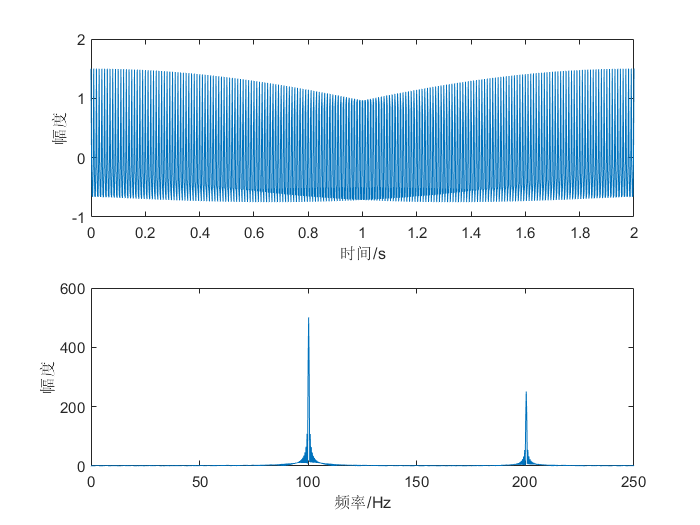可以看到，该信号由两个频率分别为100Hz和200Hz的分量构成。利用频谱分析，我们可以发现一些时域中看不到的信息，比如信号的频率分量组成、信号能量在频域上的分布等。

然而，傅里叶变换是一种全局的变换，时域信号经过傅里叶变换后，就变成了频域信号，从频域是无法看到时域信息的，我们可以从上节中的傅里叶变换和反变换公式进行解释，进行正变换时，积分区间为整个时域，所以变换结果将不包含时域信息，反变换同理。

如果信号的频率特性在任何时间都不发生改变(即该信号是平稳信号)的话，使用傅里叶变换是没有问题的，然而如果该信号是非平稳信号，这时候时域信息就相当重要了。举个栗子，以下分别是0100Hz线性递增扫频信号和1000Hz线性递减扫频信号的幅度谱，这两个信号都是非平稳信号，可以看到它们的幅度谱是相同的，很显然我们无法知道每一个频率分量出现的时间，即无法知道扫频信号的模式。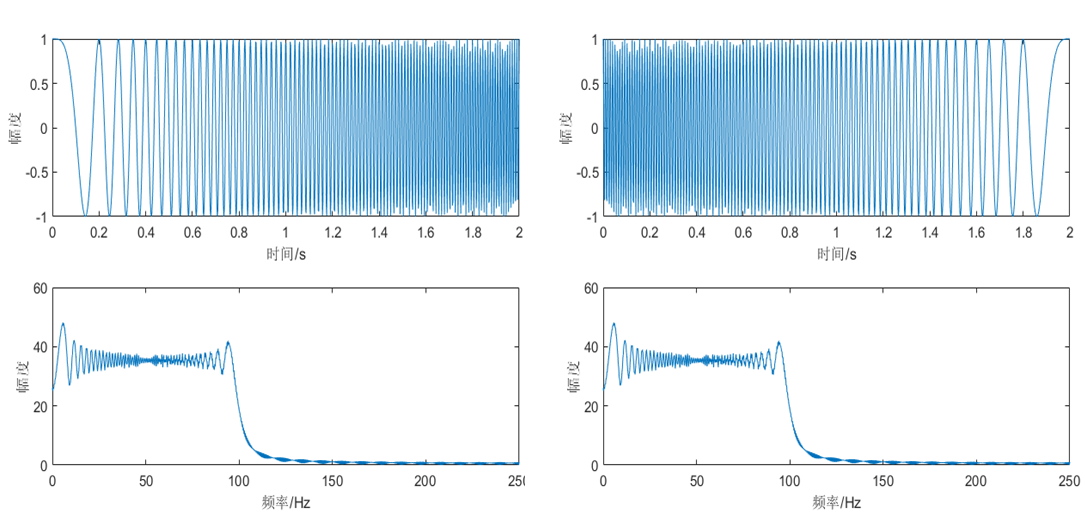上述例子可能比较简单，可以直接从时域信号看出扫频模式，但是对于一些复杂的信号和它的幅度谱，比如以下的两路扫频信号叠加后的信号和它的幅度谱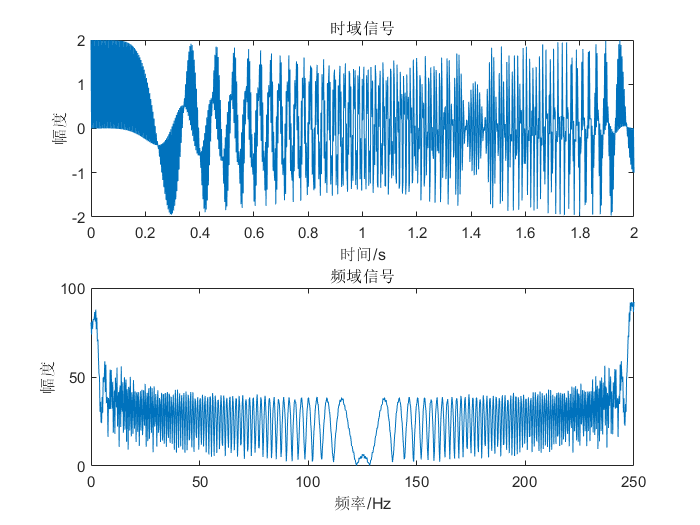仅仅通过时域信号或者幅度谱，我们是很难分析这段非平稳信号的特征的。下面我们将引入一个时频分析( Time-Frequency Analysis)方法——短时傅里叶变换(STFT)。

短时傅里叶变换(Short-Time Fourier Transform, STFT)

短时傅里叶变换定义为
X ( n , ω ) = ∑ m = − ∞ ∞ x ( m ) w ( n − m ) e − j ω m X(n,\omega)=\sum_{m=-\infty}^\infty x(m)w(n-m)e^{-j\omega m}
其中 x ( m ) x(m) 为输入信号， w ( m ) w(m) 是窗函数，它在时间上反转并且有n个样本的偏移量。 X ( n , ω ) X(n,\omega) 是时间 n n 和频率 ω \omega 的二维函数，它将信号的时域和频域联系起来，我们可以据此对信号进行时频分析，比如 S ( n , ω ) = ∣ X ( n , ω ) ∣ 2 S(n,\omega)=|X(n,\omega)|^2 就是语音信号所谓的语谱图(Spectrogram)。

画出上节中两路扫频信号叠加后的信号的语谱图，如下图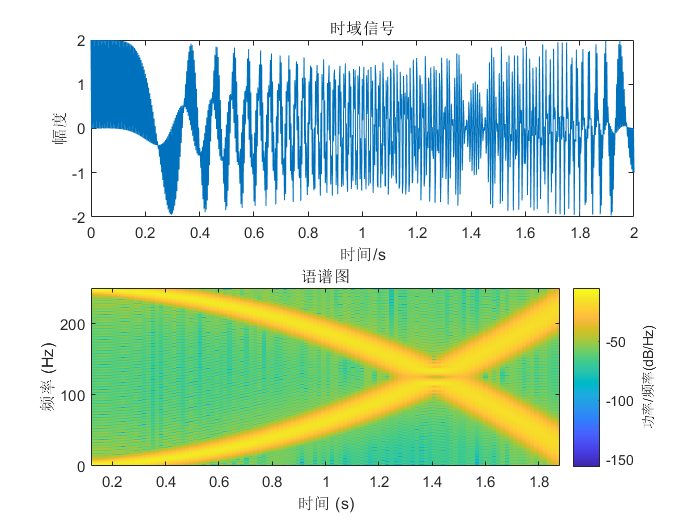可见该信号是由一个0 ~ 250Hz二次递增的扫频信号和一个250 ~ 0Hz二次递减的扫频信号的叠加。通过STFT，我们可以很容易地得出非平稳信号的时变特性。

继续对STFT进行分析。

计算语谱 S ( n , ω ) S(n,\omega) 时采用不同窗长度，可以得到两种语谱图，即窄带和宽带语谱图。长时窗（至少两个基音周期）常被用于计算窄带语谱图，短窗则用于计算宽带语谱图。窄带语谱图具有较高的频率分辨率和较低的时间分辨率，良好的频率分辨率可以让语音的每个谐波分量更容易被辨别，在语谱图上显示为水平条纹。相反宽带语谱图具有较高的时间分辨率和较低的频率分辨率，低频率分辨率只能得到谱包络，良好的时间分辨率适合用于分析和检验英语语音的发音。

如下图所示，分别为一段语音的帧长为128和512的语谱图。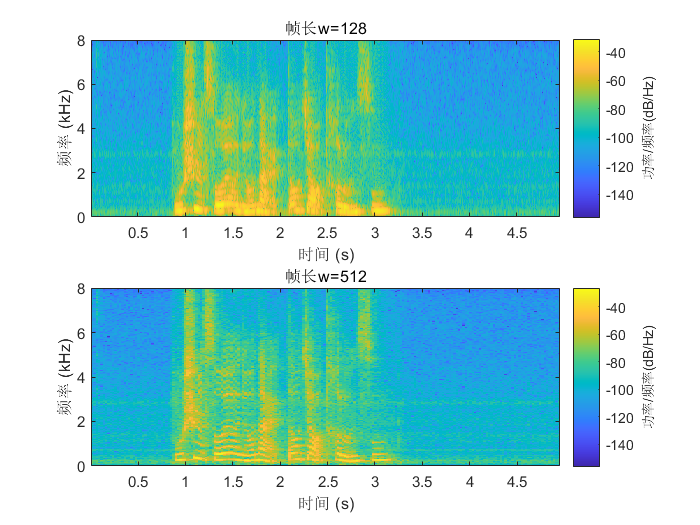可见，对于帧长固定的短时傅里叶变换，在全局范围内的时间分辨率和频率分辨率是固定的。如果我们想要在低频区域具有高频率分辨率，在高频区域具有高时间分辨率，显然STFT是不能满足要求的。我们继续引入另一种时频分析方法——小波变换。

小波变换(Wavelet Transform, WT)

对于任意能量有限信号 f ( t ) f(t) ，其连续小波变换(CWT)定义为
W f ( a , b ) = 1 a ∫ − ∞ + ∞ f ( t ) ψ ∗ ( t − b a ) d t W_f(a,b)=\frac{1}{\sqrt a}\int_{-\infty}^{+\infty}f(t)\psi^*(\frac{t-b}{a})dt
其中 ψ ( t ) \psi(t) 是母小波或者基本小波，它满足 ψ ( ± ∞ ) = 0 \psi(±\infty)=0 ψ ( 0 ) = 0 \psi(0)=0 ∫ − ∞ + ∞ ψ ( t ) d t = 0 \int_{-\infty}^{+\infty}\psi(t)dt=0 ，前两个条件表明 ψ ( t ) \psi(t) 在时域上是一个有限长的函数，最后一个条件则表明 ψ ( t ) \psi(t) 必须时正时负地波动，否则它的积分结果不会为零，因此它在频域上也是有限的。所以不同于傅里叶变换的基函数是一个无限长的正弦波，小波变换的基函数是一个经过衰减处理的有限长小波，小波基函数在时域和频域上都是局部化的，如下图分别为傅里叶变换和小波变换的基函数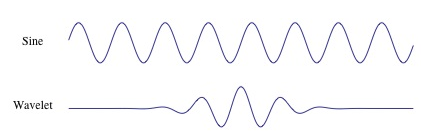ψ ( t ) \psi(t) 进行伸缩和平移得到一族函数 ψ a , b ( t ) \psi_{a,b}(t) ，称为分析小波，这就是小波变换的基函数族，其中 a a 为伸缩参数，当 a > 1 a>1 时，沿时间轴方向拉伸，因子 1 / a 1/\sqrt a 是为了保持伸缩之后能量不变； b b 为平移参数。

使用MATLAB的小波变换工具箱画出上节两路扫频信号叠加信号的小波变换结果，如下图，其中纵轴(频率轴)是对数轴。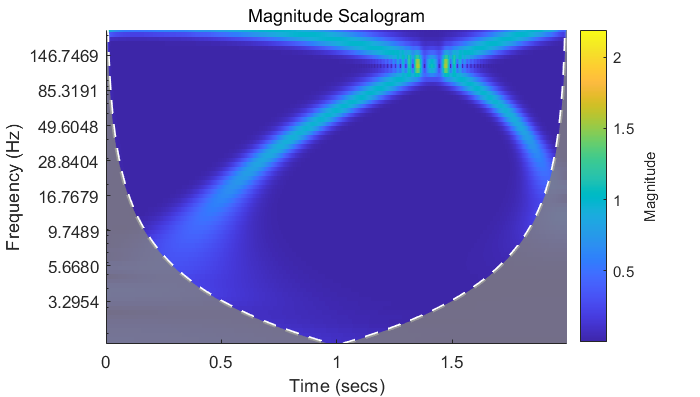可见在低频区域的变换结果具有较高的频率分辨率(频率轴是对数轴，在低频区域跨度较小)，在高频区域具有较高的时间分辨率。

一些总结

我们对上述方法进行总结，可以发现上述方法的区别都可以归结为选取的时频窗尺寸不同。画出时域信号、频域信号、使用STFT得到的时频域信号、使用小波变换得到的时频域信号的时频窗，如下图，每一个小方块表示一个时频窗，沿时间方向的边长表示时间分辨率，沿频率方向的边长表示频率分辨率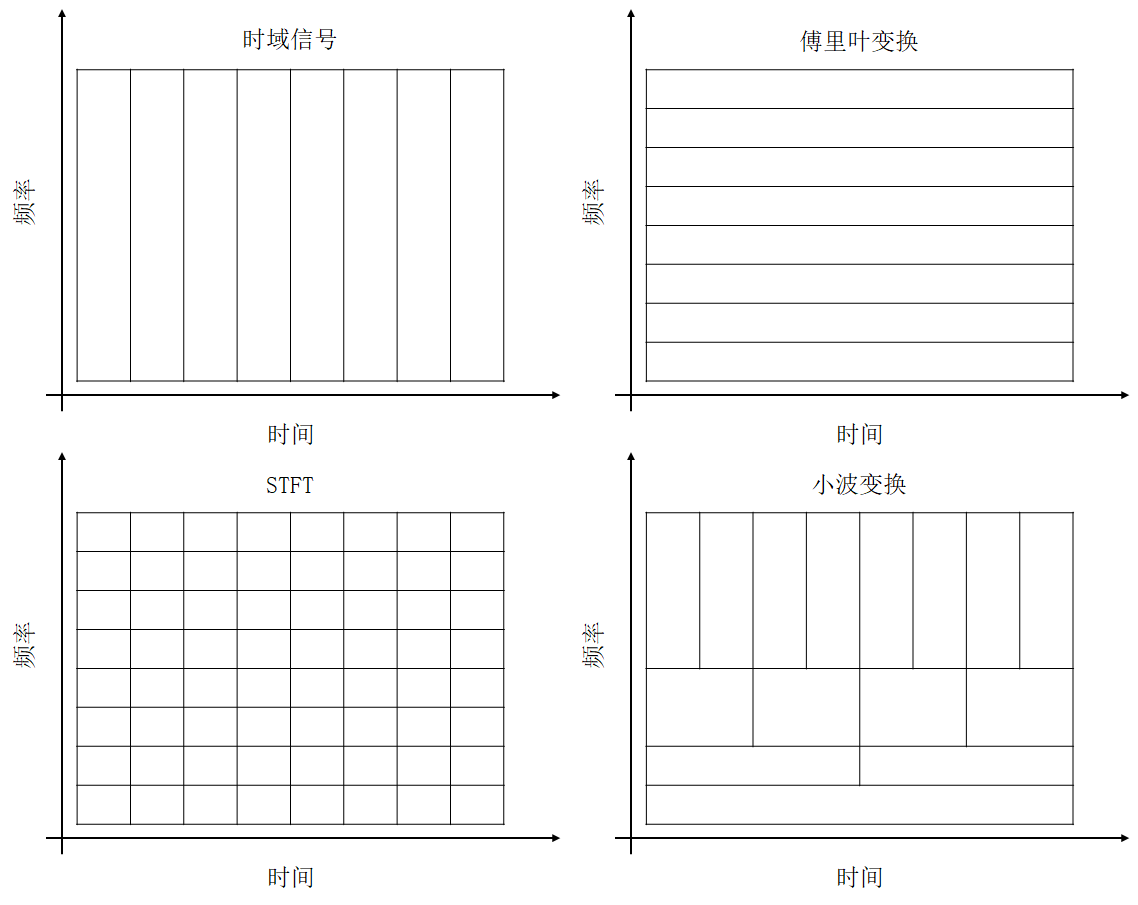由上图可以知道：

• 对于时域信号，它可以有很高的时间分辨率，然而其频率分辨率为零。

• 经过傅里叶变换得到的频域信号可以实现很高的频率分辨率，然而其时间分辨率为零。

• 对于短时傅里叶变换(STFT)，它在时域和频域都有一定的分辨率，并且在全局范围内STFT的时频分辨率都是一样的。但是由于Heisenberg不确定原理(也就是量子力学中的测不准原理)的制约，每一个时频窗的面积都是固定的，即时间分辨率和频率分辨率成反比，所以这两个分辨率不能同时很高。

• 小波变换在不同时间和频率上具有不同尺寸的时频窗，可以在低频区域实现较高的频率分辨率，然而其仍然受到Heisenberg不确定原理的限制，时间分辨率和频率分辨率不能两全其美。同时小波变换的时频窗并非完全是自适应的，它还需要人为地选择基函数。

上述的方法都会受到Heisenberg不确定原理的限制，而且并不是完全自适应的方法。接下来介绍一种不受Heisenberg不确定原理限制、同时还有更好的自适应性的时频分析方法——希尔伯特黄变换

希尔伯特变换(Hilbert Transform, HT)

在介绍希尔伯特黄变换之前，我们先介绍一下希尔伯特变换。

希尔伯特变换也是傅里叶变换的一种扩展，它常常用于通信系统中的调制解调，当然它也可以用于信号的时频分析。其计算方法为

1. 计算输入信号的FFT，保存为向量F

2. 创建一个向量h，其中
h ( i ) = { 1 , i = 1 , n 2 + 1 2 , i = 2 , 3 , ⋯   , n 2 0 , i = n 2 + 2 , ⋯   , n h(i)=\begin{cases}1&,i=1,\frac{n}{2}+1 \\2&,i=2,3,\cdots,\frac{n}{2}\\0&,i=\frac{n}{2}+2,\cdots,n\end{cases}

3. 计算F与h的内积

4. 计算上步得到的序列的iFFT

稍微介绍下它在通信系统中的应用，利用MATLAB中的hilbert函数对一个扫频信号进行希尔伯特变换，得到的结果是一个解析信号，在同一坐标系画出该解析信号的实数部分和虚数部分，如下图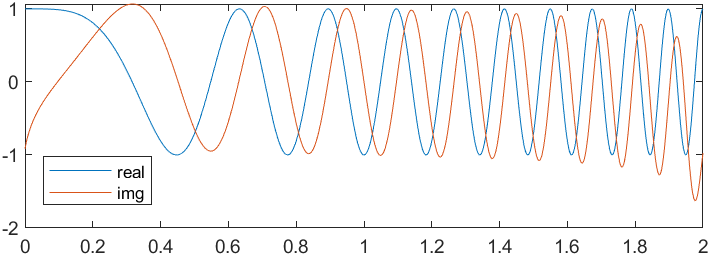可见虚数部分较实数部分滞后 π / 2 \pi / 2 ，我们可以利用这个性质消掉负频率。使用MATLAB中的fft函数对一个信号进行傅里叶变换，结果中的负频率保存在下半轴。如下是一个扫频信号的幅度谱以及使用希尔伯特变换得到的解析信号的幅度谱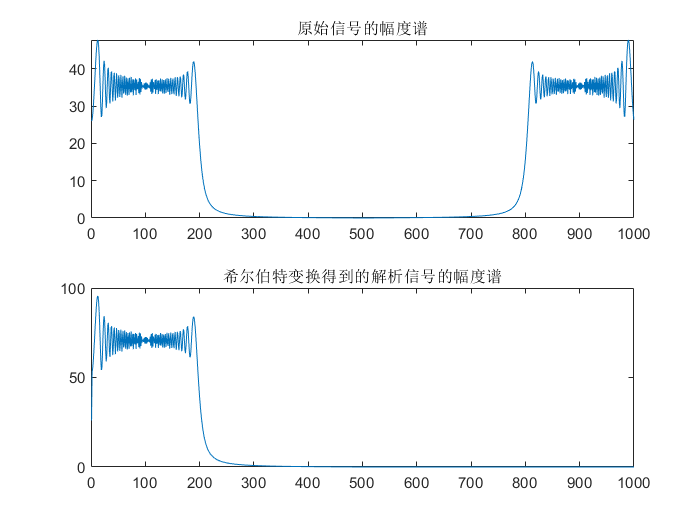由上图可见，解析信号的幅度谱没有负频率，并且各个频率分量的幅度是原来实信号的两倍。

通过希尔伯特变换可以得到没有负频率的解析信号，基于此可以实现信号的单边带调制，从而节省带宽和降低发射功率。感兴趣可以继续查看使用希尔伯特变换进行单边带幅度调制

继续探讨基于希尔伯特变换的时频分析。在时频分析领域，希尔伯特变换主要用于瞬时频率估计。

由上述分析可知使用希尔伯特变换可以得到原始信号的解析信号，假设解析信号为 z ( t ) z(t)
z ( t ) = c ( t ) + j y ( t ) = a e j θ ( t ) z(t)=c(t)+jy(t)=ae^{j\theta(t)}
其中 a ( t ) = c ( t ) 2 + y ( t ) 2 a(t)=\sqrt{c(t)^2+y(t)^2} ，表示瞬时幅值， θ ( t ) = a r c t a n y ( t ) c ( t ) \theta(t)=arctan\frac{y(t)}{c(t)} ，表示瞬时相位， ω = d θ ( t ) d t \omega=\frac{d\theta(t)}{dt} ，表示瞬时频率。由瞬时幅值和瞬时频率可将信号表示为
x ( t ) = a ( t ) e j ∫ ω ( t ) d t x(t)=a(t)e^{j\int\omega(t)dt}
若使用 ∣ a ( t ) ∣ 2 |a(t)|^2 表示瞬时能量，则可在时间-频率面上画出信号的瞬时能量分布，这个分布谱图就是Hilbert谱，记为 H ( ω , t ) H(\omega,t) 。如下为一个0~100Hz扫频信号的时域信号和它的Hilbert谱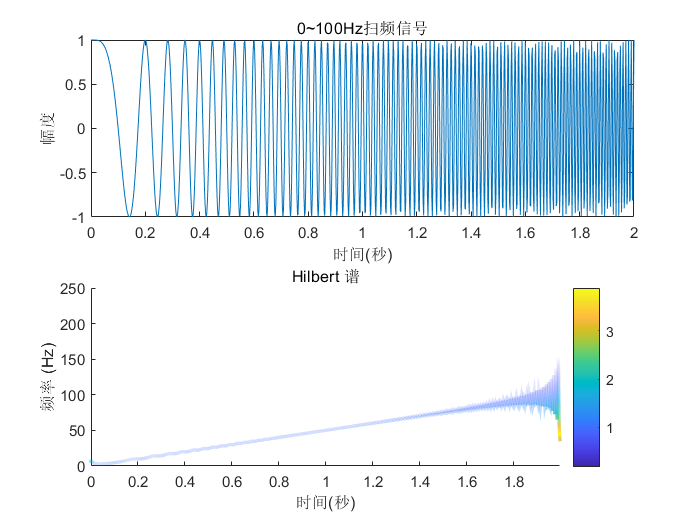上图中的信号为单频率成分信号，即同一时刻只有一个频率分量的信号，我们可以由Hilbert谱很好地观察出信号的时频特征，且有很高的的时间分辨率，但是信号边界处的误差往往较大。

如果是对一个多频率成分信号(同一时刻有多个频率分量的信号)进行希尔伯特变换，结果会怎样呢？如下为两路扫频信号叠加信号的Hilbert谱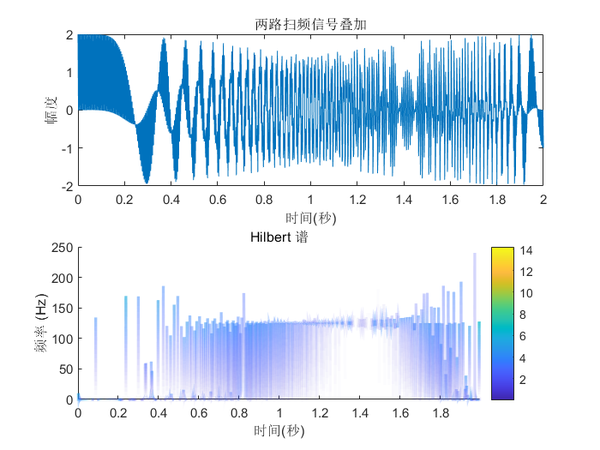从上图中的Hilbert谱我们并不能概括出该信号的时频特征，所以对多频率成分信号不能直接进行Hilbert变换，我们还需要对其进行进一步处理，将原始信号分解成单频率信号的叠加，这就要用到希尔伯特黄变换中的EMD分解。

希尔伯特黄变换(Hilbert-Huang Transform, HHT)

相比于HT，HHT就多了一个经验模态分解(Empirical Mode Decomposition, EMD)，EMD就是把复杂信号分解成从高频到低频的若干个固有模态函数(Intrinsic Mode Function, IMF)，IMF需要满足两个条件：

1. 信号极值点的数量与零点数相等或相差为1
2. 信号的由极大值定义的上包络和由极小值定义 的下包络的局部均值为0(即包络上下对称)

简单的理解就是，EMD是依次提取信号在每个局部的最高频分量的过程，所以每个IMF实际上是一个单频率分量信号，这样我们就可以对每个IMF分量进行Hilbert变换，从而得到每个分量的Hilbert谱。如下是对两个扫频信号叠加后的信号进行EMD分解得到的IMF分量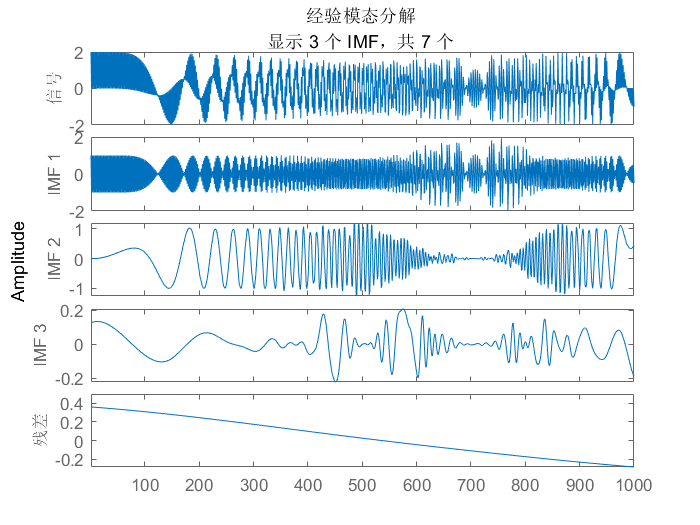对上述的IMF分量进行Hilbert变换，求得Hilbert谱，如下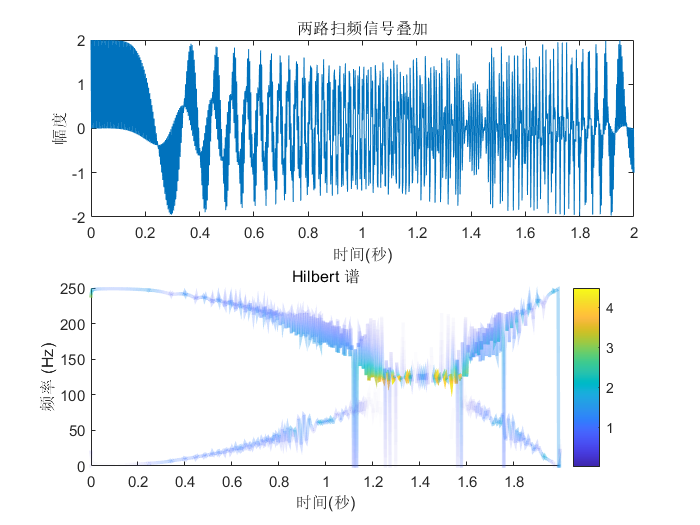从上图我们大致可以看出信号的时频特性：该信号是一个0 ~ 250Hz二次递增的扫频信号和一个250 ~ 0Hz二次递减的扫频信号的叠加。

当然HHT并不是完美的，目前对于它的关键步骤EMD分解的研究尚不完善，缺乏一些理论基础。从上图我们也可以看到，HHT在低频区域可能会出现一些不存在的频率分量。

参考

https://blog.csdn.net/Forlogen/article/details/88535027

https://www.sohu.com/a/246972969_607269

唐晓初. 小波分析及其应用[M]. 重庆大学出版社, 2006.

Marple L . Computing the discrete-time “analytic” signal via FFT[J]. IEEE Transactions on Signal Processing, 1999.

罗利春. 用希尔伯特变换构造解析信号进行时频分析[J]. 航天电子对抗, 2003(03):27-30.

Qian S , Chen D . Joint time-frequency analysis[J]. IEEE Signal Processing Magazine, 1999, 16(2): P.52-67.

展开全文qq_42688495 2020-06-25 21:06:10
• 希尔伯特黄变换(HHT)的 完整 MATLAB程序matlab2021-2-12下载地址https://www.codedown123.com/64242.html希尔伯特黄变换(HHT)的 完整 MATLAB程序资源下载此资源下载价格为2D币，请先登录资源文件列表╫ε║≤▓Γ╩...

希尔伯特黄变换(HHT)的 完整 MATLAB程序

matlab

2021-2-12

下载地址

https://www.codedown123.com/64242.html

希尔伯特黄变换(HHT)的 完整 MATLAB程序

资源下载此资源下载价格为2D币，请先登录

资源文件列表

╫ε║≤▓Γ╩╘/333.jpg , 201517

╫ε║≤▓Γ╩╘/FAacos.m , 986

╫ε║≤▓Γ╩╘/FAcosfor.m , 1129

╫ε║≤▓Γ╩╘/FAhilbert.m , 401

╫ε║≤▓Γ╩╘/FAimpHilbert.m , 371

╫ε║≤▓Γ╩╘/FAzc.m , 2553

╫ε║≤▓Γ╩╘/FSPHSP.m , 1660

╫ε║≤▓Γ╩╘/LOD78.csv , 29021

╫ε║≤▓Γ╩╘/blocknormalize.m , 1203

╫ε║≤▓Γ╩╘/confidenceLine.m , 1078

╫ε║≤▓Γ╩╘/dist_value.m , 310

╫ε║≤▓Γ╩╘/duang.m , 1121

╫ε║≤▓Γ╩╘/eemd.m , 1180

╫ε║≤▓Γ╩╘/emax.m , 222

╫ε║≤▓Γ╩╘/emin.m , 220

╫ε║≤▓Γ╩╘/endprocess1.m , 5184

╫ε║≤▓Γ╩╘/extrema.m , 1616

╫ε║≤▓Γ╩╘/fa.m , 2875

╫ε║≤▓Γ╩╘/findEE.m , 2345

╫ε║≤▓Γ╩╘/findEEfsp.m , 5891

╫ε║≤▓Γ╩╘/findcriticalpoints.m , 1307

╫ε║≤▓Γ╩╘/fspecial.m , 7476

╫ε║≤▓Γ╩╘/gui17.fig , 1241

╫ε║≤▓Γ╩╘/gui17.m , 2991

╫ε║≤▓Γ╩╘/hilbert.m , 456

╫ε║≤▓Γ╩╘/hilbertnormalize.m , 415

╫ε║≤▓Γ╩╘/hilbtm.m , 9731

╫ε║≤▓Γ╩╘/ifndq.m , 1340

╫ε║≤▓Γ╩╘/linearnormalize.m , 1056

╫ε║≤▓Γ╩╘/local_max.m , 979

╫ε║≤▓Γ╩╘/medianfilter.m , 157

╫ε║≤▓Γ╩╘/nnspe.m , 1587

╫ε║≤▓Γ╩╘/nspplote.m , 2635

╫ε║≤▓Γ╩╘/pchipnormalize.m , 1032

╫ε║≤▓Γ╩╘/ratio1.m , 202

╫ε║≤▓Γ╩╘/ratioa.m , 199

╫ε║≤▓Γ╩╘/secondtime.fig , 9824

╫ε║≤▓Γ╩╘/secondtime.m , 8519

╫ε║≤▓Γ╩╘/significanceIMF.m , 764

╫ε║≤▓Γ╩╘/signiplotIMF.m , 1126

╫ε║≤▓Γ╩╘/skiphilbt_m.m , 209

╫ε║≤▓Γ╩╘/splinenormalize.m , 1047

╫ε║≤▓Γ╩╘/splinenormalizeep.m , 1347

╫ε║≤▓Γ╩╘/untitled1.fig , 6404

╫ε║≤▓Γ╩╘/untitled1.m , 5263

展开全文weixin_34379989 2021-04-26 18:26:56
• 希尔伯特黄变换代码 matlab

23KB qq_17025315 2019-03-25 17:18:36
• 1.08MB weixin_45928487 2020-05-27 09:53:48
• 5.9MB qq_26984409 2018-07-12 22:10:11
• 希尔伯特黄变换工具箱 希尔伯特黄

101KB liu6819182 2017-12-28 21:11:47
•5星
2.89MB qq_31747775 2018-05-02 09:23:23
•3星
918KB whapril 2011-03-16 23:00:10
• 2.1MB weixin_38624519 2021-04-16 15:33:27
• 信号处理：希尔伯特黄变换 信号处理 希尔伯特黄变换 经验模态分解

目录： 目录： 前言 简介 基本原理 经验模态分解 希尔伯特变换 ...(1)HHT能分析非线性非平稳信号。...(3)HHT不受Heisenberg测不准原理制约——适合突变信号。...最近在做信号处理，发现自己基本功不够...希尔伯特黄变换 ...

前言

最近在做信号处理，发现自己基本功不够扎实，开始了恶补之路，希望能够尽快的补齐吧。

简介

希尔伯特黄变换
1998年，Norden E. Huang（黄锷：中国台湾海洋学家）等人提出了经验模态分解方法，并引入了Hilbert谱的概念和Hilbert谱分析的方法，美国国家航空和宇航局（NASA）将这一方法命名为Hilbert-Huang Transform，简称HHT，即希尔伯特-黄变换
HHT主要内容包含两部分，第一部分为经验模态分解（Empirical Mode Decomposition，简称EMD），它是由Huang提出的；第二部分为Hilbert谱分析（Hilbert Spectrum Analysis，简称HSA）。简单说来，HHT处理非平稳信号的基本过程是：首先利用EMD方法将给定的信号分解为若干固有模态函数（以Intrinsic Mode Function或IMF表示，也称作本征模态函数），这些IMF是满足一定条件的分量；然后，对每一个IMF进行Hilbert变换，得到相应的Hilbert谱，即将每个IMF表示在联合的时频域中；最后，汇总所有IMF的Hilbert谱就会得到原始信号的Hilbert谱。

基本原理

经验模态分解

经验模态分解往往被称为是一个“筛选”过程。这个筛选过程依据信号特点自适应地把任意一个复杂信号分解为一系列本征模态函数(IntrinsicMode Function, IMF)。它满足如下两个条件：
(1) 信号极值点的数量与零点数相等或相差是一；
(2) 信号的由极大值定义的上包络和由极小值定义的下包络的局部均值为零。
EMD 筛选过程如下：
(1) 对输入信号 x(t) x ( t ) $x(t)$，求取极大值点 x(ti) x ( t i ) $x(t_i)$，和极小值点 x(tj) x ( t j ) $x(t_j)$ xu(t) x u ( t ) $x_u(t)$
(2) 对极大值点和极小值点采用三次样条函数插值构造信号上下包络、 xl(t) x l ( t ) $x_l(t)$, 计算上、下包络的均值函数；
(3) 考察是否满足IMF 条件，如果满足则转到下一步，否则对 xl x l $x_l$进行前两步操作,求 m11 m 11 $m_{11}$
得以及，依次下去，直到第k步满足IMF条件，则求得第一个IMF ；
(4) 得到第一个残留，对作如同上述三步操作，得到 c2 c 2 $c_2$
以及以此类推；
(5) 直到 rn r n $r_n$为单调信号或者只存在一个极点为止。原始信号被表达为。

希尔伯特变换

这类本征模态函数的瞬时频率(Instantaneous Frequency ,IF)有着明确的物理意义。因此,经验模态分解后, 对每一个IMF作希尔伯特变换( Hilbert Transform,HT)，继而可求取每一个IMF的瞬时频率。
对任意信号x(t)，称为x(t)的希尔伯特变换,其中P.V表示Cauchy 主值积分。
通过HT，可以构造解析信号z(t)，并在极坐标下表达为: ，其中，，则x(t)的瞬时频率定义为。
综合上述两步,原信号表达为，为一个时间-频率-能量三维分布图。

特点

与传统的信号或数据处理方法相比，HHT具有如下特点：

(1)HHT能分析非线性非平稳信号。

传统的数据处理方法，如傅立叶变换只能处理线性非平稳的信号，小波变换虽然在理论上能处理非线性非平稳信号，但在实际算法实现中却只能处理线性非平稳信号。历史上还出现过不少信号处理方法，然而它们不是受线性束缚，就是受平稳性束缚，并不能完全意义上处理非线性非平稳信号。HHT则不同于这些传统方法，它彻底摆脱了线性和平稳性束缚，其适用于分析非线性非平稳信号。

(2)HHT具有完全自适应性。

HHT能够自适应产生“基”，即由“筛选”过程产生的IMF。这点不同于傅立叶变换和小波变换。傅立叶变换的基是三角函数，小波变换的基是满足“可容性条件”的小波基，小波基也是预先选定的。在实际工程中，如何选择小波基不是一件容易的事，选择不同的小波基可能产生不同的处理结果。我们也没有理由认为所选的小波基能够反映被分析数据或信号的特性。

(3)HHT不受Heisenberg测不准原理制约——适合突变信号。

傅立叶变换、短时傅立叶变换、小波变换都受Heisenberg测不准原理制约，即时间窗口与频率窗口的乘积为一个常数。这就意味着如果要提高时间精度就得牺牲频率精度，反之亦然，故不能在时间和频率同时达到很高的精度，这就给信号分析处理带来一定的不便。而HHT不受Heisenberg测不准原理制约，它可以在时间和频率同时达到很高的精度，这使它非常适用于分析突变信号。

(4)HHT的瞬时频率是采用求导得到的。

傅立叶变换、短时傅立叶变换、小波变换有一个共同的特点，就是预先选择基函数，其计算方式是通过与基函数的卷积产生的。HHT不同于这些方法，它借助Hilbert变换求得相位函数，再对相位函数求导产生瞬时频率。这样求出的瞬时频率是局部性的，而傅立叶变换的频率是全局性的，小波变换的频率是区域性的。

展开全文lvsehaiyang1993 2018-07-01 10:50:23
• 【参考资料】 【1】https://www.cnblogs.com/qiweiwang/archive/2010/12/20/1911736.html...【4】《希尔伯特变换与信号的包络_瞬时相位和瞬时频率》 https://wenku.baidu.com/view/9aa65710580216fc700afd57.html 1. 概

【参考资料】
【1】https://www.cnblogs.com/qiweiwang/archive/2010/12/20/1911736.html
【2】https://zhuanlan.zhihu.com/p/74413218
【3】https://www.pianshen.com/article/9161269068/
【4】《希尔伯特变换与信号的包络_瞬时相位和瞬时频率》 https://wenku.baidu.com/view/9aa65710580216fc700afd57.html

1. 概述

所谓HHT 希尔伯特黄变换是将信号进行EMD分解，将分解完的每个IMF分量用Hibert变换得到其瞬时频谱，最终进行信号的时频分析。因此在本笔记中重点记录hilbert变换和EMD分解。

2. Hilbert变换与瞬时频率

2.1 复信号的定义

已知有欧拉公式：

e j θ = c o s θ + j s i n θ e^{j \theta} = cos \theta + j sin \theta

由公式 θ = ω t = 2 π T t \theta = \omega t = \dfrac{2 \pi}{T}t 得到下述复变函数公式：

e j ω = c o s ω t + j s i n ω t e^{j\omega} = cos \omega t + j sin \omega t 从而反推得到：

c o s ω t = e j ω + e − j ω cos \omega t = e^{j\omega} + e^{-j\omega}

由于上面和式中如果根据欧兰公式展开，其虚部是互相抵消的，因此我们可以将实信号表示成：

c o s ω t = R e s { e j ω } cos \omega t = Res\{ e^{j\omega} \} ,即 e j ω e^{j\omega} 这个信号的实部，此时我们称为 e j ω e^{j\omega} 为其复信号

2.2 实信号和复信号的频谱关系

推导详见【参考文献4】，结论为：

q ( t ) q(t) x ( t ) x(t) 的复信号，其中 Q ( f ) Q(f) X ( f ) X(f) 分别为其频谱，这有：

Q ( f ) = { 2 Q ( f ) f > 0 0 f < 0 Q(f)=\left\{ \begin{aligned} 2Q(f)& & f > 0 \\ 0 & & f < 0 \end{aligned} \right.

针对这种关系，我们可以定义一个滤波器,其频谱为 H 1 ( f ) = { 2 f > 0 0 f < 0 H_1(f)=\left\{ \begin{aligned} 2 & & f > 0 \\ 0 & & f < 0 \end{aligned} \right.

同时得到其对应的时间函数： h 1 ( t ) = δ ( t ) + i 1 π t h_1(t) = \delta(t) + i \dfrac{1}{\pi t}
备注：这步不知道怎么推出来的?

2.3 Hilbert变换的定义

由2.2存在公式（频域的乘法为时域的卷积），我们有：

q ( t ) = h 1 ( t ) ∗ x ( t ) q(t) = h_1(t) * x(t)

q ( t ) = （ δ ( t ) + i 1 π t ） ∗ x ( t ) q(t) = （\delta(t) + i \dfrac{1}{\pi t}） * x(t) 得到

q ( t ) = x ( t ) + i 1 π t ∗ x ( t ) q(t) = x(t) + i \dfrac{1}{\pi t} * x(t)

x ~ ( t ) = 1 π t ∗ x ( t ) = 1 π ∫ − ∞ ∞ x ( τ ) t − τ d τ \widetilde{x}(t) = \dfrac{1}{\pi t} * x(t) = \dfrac{1}{\pi} \int_{-\infty}^{\infty} \dfrac{x(\tau)}{t - \tau} d \tau

我们称 x ~ ( t ) \widetilde{x}(t) 为Hilbert变换

这里的Hilbert变换相当于做了一个滤波，频谱滤波为： H 1 ( f ) = { i f > 0 − i f < 0 H_1(f)=\left\{ \begin{aligned} i & & f > 0 \\ -i & & f < 0 \end{aligned} \right.

举例：2.4 解析信号、包络、瞬时相位和瞬时频谱

利用实信号 x ( t ) x(t) 及其hilbert变换 x ~ ( t ) \widetilde{x}(t) 构成一个解析信号

q ( t ) = x ( t ) + x ~ ( t ) q(t) = x(t) + \widetilde{x}(t)

假设有一工程中常用的窄带信号：

x ( t ) = a ( t ) c o s ( ω t + φ ( t ) ) x(t) = a(t) cos(\omega t + \varphi(t)) 及其hilbert变换 x ~ ( t ) = a ( t ) s i n ( ω t + φ ( t ) ) \widetilde{x}(t) = a(t) sin(\omega t + \varphi(t)) 推导详见参考文献4 略

我们令 θ ( t ) = ω t + φ ( t ) \theta(t) = \omega t + \varphi(t) 则有如下一些核心定义：

包络： e ( t ) = x ( t ) 2 + x ~ ( t ) 2 e(t) = \sqrt{x(t)^2 + \widetilde{x}(t)^2}

瞬时相位： θ ( t ) = a r c t g x ~ ( t ) x ( t ) \theta(t) = arctg \dfrac{\widetilde{x}(t)}{x(t)}

瞬时频率： u ( t ) = d θ ( t ) d t = d d t a r c t g x ~ ( t ) x ( t ) u(t) = \dfrac{d \theta (t)}{dt} = \dfrac{d}{dt} arctg \dfrac{\widetilde{x}(t)}{x(t)} 是瞬时相位的导数

备注：F(t) = sin(2πft + φ)：f就是频率；2πft + φ 就是相位；对时间的导数就是2πf，频率是相位的变换速度

2.5 python代码
import numpy as np
import pylab as pl
import scipy.signal as signal
import matplotlib.pyplot as plt
from scipy import fftpack
import math
from tftb.processing import inst_freq

t     = np.arange(0, 0.1, 1/20000.0)
x     = 4*t*np.sin(2*np.pi*200*t + 3.0) + 0.1
hx    = fftpack.hilbert(x)
e     = np.sqrt(x**2 + hx**2)
u1, _ = inst_freq(hx)

t = []
for i in range(len(x)):
t.append(math.atan(hx[i]/x[i]))

u = []
for i in range(len(t)-1):
u.append(t[i+1] - t[i])

plt.figure(figsize=(10,7))
plt.subplot(3, 1, 1)
plt.plot(x,'r') #hilbert变换
plt.subplot(3, 1, 1)
plt.plot(hx,'g') #hilbert变换
plt.subplot(3, 1, 1)
plt.plot(e,'b') #包络曲线
plt.subplot(3, 1, 2)
plt.plot(t,'r') #瞬时相位
plt.subplot(3, 1, 3)
plt.plot(u,'r') #瞬时频率
plt.show()备注：这里是按照自己的理解去实现离散Hilbert变换的瞬时相位和频率，但和 inst_freq 似乎不太对？？？

3. 经验模态分解EMD分解

3.1 EMD分解的基本步骤：

步骤1：

1. 找出原始信号 x ( t ) x(t) 局部极大值，用三次B样条构筑上包络曲线
2. 找出原始信号 x ( t ) x(t) 局部极小值，用三次B样条构筑下包络曲线步骤2：

1. 计算上包络曲线和下包络曲线的均值 m 1 m_1
2. 计算原始曲线与包络均值的差： h 1 = x ( t ) − m 1 h_1 = x(t) - m_1

步骤3：

1. 判断 h 1 h_1 是否满足IMF条件

备注：IMF条件需要满足两点：
2.1 经过分解得到的时间序列，其极值点数量与过0点数量相差不超过1个
2.2 经过插值方式拟合的极值包络线在分析时间序列内的均值为0

1. 如果不满足IMF条件，这重复步骤1、步骤2，使得k步迭代后的 h 1 , k ( t ) h_{1,k}(t) 作为第一个IMF分量 c 1 ( t ) = h 1 , k ( t ) c_1(t)=h_{1,k}(t)

步骤4：

1. 计算剩余信号 r 1 ( t ) = x ( t ) − c 1 ( t ) r_1(t) = x(t) - c_1(t)

步骤5：

1. 针对剩余信号 r ( t ) r_(t) 重复步骤1~3,求得其余的IMF分量 r ( t ) … r N ( t ) r_(t) \dots r_{N}(t)
2. 最终原始信号被分解为IMF分量和剩余信号的和，即 x ( t ) = ∑ n = 1 N c n ( t ) + r N ( t ) x(t) = \sum_{n=1}^N c_n(t) + r_N(t)

备注：在其他资料中，步骤3的k步迭代是否满足IMF条件，采用公式：要求SD的值在0.2~0.3之间

3.2 python代码

import pyhht
from pyhht.visualization import plot_imfs

t = np.arange(0, 0.1, 1/20000.0)
x = 4*t*np.sin(2*np.pi*200*t + 3.0) + 0.1
decomposer = pyhht.emd.EMD(x)
imfs = decomposer.decompose()
plot_imfs(x, imfs)展开全文Fredric_2014 2020-11-01 14:38:51
• 22KB leavemyleave 2021-10-15 01:09:01
• EMD-希尔伯特黄变换 EMD 希尔伯特黄

20KB polweat 2015-07-20 21:47:30
• 356KB weixin_42320441 2018-08-13 18:30:52...